```

```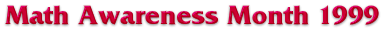# Periodic Functions

You may have noticed the common mathematical thread which runs through all blood-flow related phenomena: they all repeat over and over. The heart goes through its cycle of contraction and relaxation (called a systole), performing the same proccess 70 times a minute. Pressure also goes up and down repeatedly, and so does blood flow - when the heart contracts, pressure rises, when the heart relaxes (for a split second) the pressure drops. This behavior is known as periodic, and mathematicians have special functions that describe this behavior - they are sines and cosines that we learn in trigonometry (see figure).In fact, blood flows through arteries, very much like a sine wave, where the amplitude is the maximum blood flow, and the frequency is the heart rate. There is a beatiful partial differential equation known as the wave equation, which describes an idelized wave, be it ocean waves, blood flowing periodically, or ripples spreading in a pond from the place where you dropped a pebble.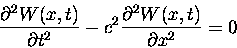One can make a few comments about this equation, without solving it just yet. What we mean by solving this equation is finding a function W of both x and t, which satisfies the equation. This function, in our application, denotes the quantity of blood passing through a point in the artery at a given moment. We also see that equation involves the second derivative in time (t) and the second derivative in space (x), which should suggest that the solution should look similar in time and in space. There is also one constant c present in the equation, which happens to be the speed with which the wave propagates. The constant is determined by the environment: how hard the heart is pumping, how thick your blood is, etc.

We would like to find solutions of the wave equation that we see in nature - the sine and cosine waves. Below I will outline the steps that are necessary to solve this old chestnut, and for more details, just click on the links.

• Use separation of variables to reduce the PDE to two ODEs, which are: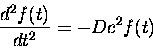• Solve the ODEs to obtain periodic solutions: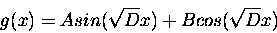• Apply the boundary conditions to obtain the solution:This function is a reasonably realistic model for blood flow in the arteries (not the capillaries, though). Notice that the frequency in time is ten times the frequency in space, which corresponds to our chosen speed of propagation of 10 cm/sec. The solution is the cosine wave in space that moves through time, like this: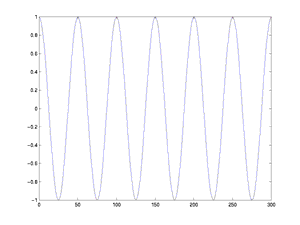```

```

M & B Exhibits | intro | pg 2 | pg 3 | pg 4 | pg 5 | pg 6 | pg 7 | pg 8